085-1263404

### My Journal

All things Mathematical
19 Aug 2018
##### Question 1

Being given a small nearly frictionless truck of known weight, a weight of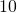lb.,small weights of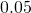lb. each, and a horizontal plane fitted with pulley, describe in detail how you would show experimentally that the acceleration of a system of constant mass is proportional to the force producing the acceleration.

##### Question 2

The amplitude of the vibration of a simple pendulum, of lengthft, making small oscillations is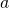ft. Find the maximum velocity of the bob.

A bullet weighingoz. is fired horizontally into a block weighing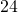lb. suspended as a ballistic pendulum of lengthfeet and becomes embedded in it. If the block is displaced through a horizontal distance of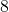inches, find (i) the velocity of the block immediately after the impact, (ii) the velocity of the shot immediately before it struck the block.

##### Question 3

A man standing on a train which is moving at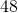miles per hour shoots at an object which is moving away from the railway track at right angles with a speed of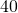miles per hour. If the bullet, which is supposed to move in a horizontal straight line, has a velocity of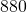ft. per second and if the line connecting the man and object makes an angle of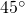with the direction in which the train is moving, find at what angle to the train he must aim in order to hit the the object.

##### Question 4

A sphere weighing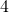lb. is suspended by a light, inextensible wire so that its c.g. isft. below the point of support. It is projected with an initial horizontal velocity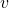so that the wire ceases to  be taut when it makes an angle of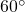with the upward-drawn vertical. Find the velocity of projection and the greatest vertical height attained above the point of projection.

##### Question 5

A cycle track has a radius of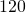ft. and is to be banked for a speed of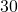miles per hour. Determine the angle which the track surface must make with the horizontal (i) when the friction between the wheels and the surface is entirely neglected, (ii) when the co-efficient of friction is taken as having a value of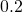.

##### Question 6

Taking the frictional resistance as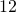lb. per ton, find the Horse Power required to produce a speed of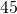miles per hour in a train weighing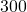tons inminutes (i) on the level, (ii) down an incline ofin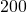.

##### Question 7

A pin-jointed framework, of the form shown in the diagram, is loaded as indicated. It is supported by a fixed pin at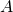and is kept in position with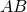vertical by a horizontal force at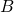. Determine the stresses in the bars, indicating in each case whether the bar is thrust or in tension.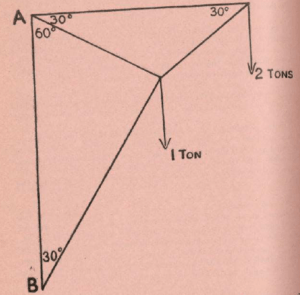##### Question 8

Show that the total work done in raising a body against gravity by any part is equal to the product of the weight and the vertical height through which the c.g. is raised. Does this principle hold if the body is not rigid? A chain weighinglb. per foot length and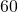ft. long hangs over a pulley with one endft. above the other. Find the work done in bringing the lower end to withinft. of the level of the other end.

##### Question 9

Explain what is meant by “the angle of friction.” Of a body be placed on a rough horizontal table show that no thrust, however great, applied to the body at an angle with the normal to the plane less than the angle of friction, can push the boy along the plane.

A ladderfeet long, weighing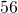lb., has one end resting on a concrete floor and the other against a vertical wall. The c.g. isft. from the lower end, and a man weighing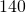lb. stands on a rung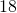ft. from the same end. If the co-efficient of friction at each end is, find the inclination of the ladder if it is just about to slip.

##### Question 10

A ball weighinglb. describes a horizontal circle, the ball being attached to two cords, the other ends of which are attached to two points in the same vertical line. Each of these points is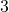feet from the centre of the ball and the cords are at right angles. If the ball makesrevolutions per second, find the tension of each cord in lbs.

##### Citation:

State Examinations Commission (2018). State Examination Commission. Accessed at: https://www.examinations.ie/?l=en&mc=au&sc=ru

Malone, D and Murray, H. (2016). Archive of Maths State Exams Papers. Accessed at: http://archive.maths.nuim.ie/staff/dmalone/StateExamPapers/

##### Licence:

“Contains Irish Public Sector Information licensed under a Creative Commons Attribution 4.0 International (CC BY 4.0) licence”.

The EU Directive 2003/98/EC on the re-use of public sector information, its amendment EU Directive 2013/37/EC, its transposed Irish Statutory Instruments S.I. No. 279/2005, S.I No. 103/2008, and S.I. No. 525/2015, and related Circulars issued by the Department of Finance (Circular 32/05), and Department of Public Expenditure and Reform (Circular 16/15 and Circular 12/16).

Note. Circular 12/2016: Licence for Re-Use of Public Sector Information adopts CC-BY as the standard PSI licence, and notes that the open standard licence identified in this Circular supersedes PSI General Licence No: 2005/08/01.